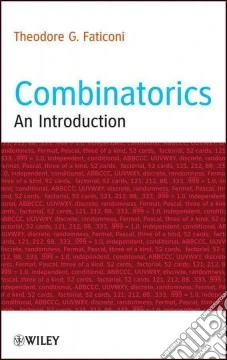ricerca
avanzata

# Combinatorics

## Un libro in lingua di Faticoni Theodore G. edito da John Wiley & Sons Inc, 2013

• € 86,20
• Il prezzo è variabile in funzione del cambio della valuta d’origineBridges combinatorics and probability and uniquely includes detailed formulas and proofs to promote mathematical thinking

Combinatorics: An Introduction introduces readers to counting combinatorics, offers examples that feature unique approaches and ideas, and presents case-by-case methods for solving problems.

Detailing how combinatorial problems arise in many areas of pure mathematics, most notably in algebra, probability theory, topology, and geometry, this book provides discussion on logic and paradoxes; sets and set notations; power sets and their cardinality; Venn diagrams; the multiplication principal; and permutations, combinations, and problems combining the multiplication principal. Additional features of this enlightening introduction include:

• Worked examples, proofs, and exercises in every chapter
• Detailed explanations of formulas to promote fundamental understanding
• Promotion of mathematical thinking by examining presented ideas and seeing proofs before reaching conclusions
• Elementary applications that do not advance beyond the use of Venn diagrams, the inclusion/exclusion formula, the multiplication principal, permutations, and combinations

Combinatorics: An Introduction is an excellent book for discrete and finite mathematics courses at the upper-undergraduate level. This book is also ideal for readers who wish to better understand the various applications of elementary combinatorics.